# How to calculate the arc length and string height of the curved LED display?

Generally, the conventional LED display screen is used, and the curved screen is used less, and the curved screen must know the arc length, the chord length, the string height, etc., and can calculate the area of â€‹â€‹the steel structure and the curved screen, etc. The display manufacturer Tuosheng Optoelectronics gives you a detailed introduction to the calculation method of the curved screen.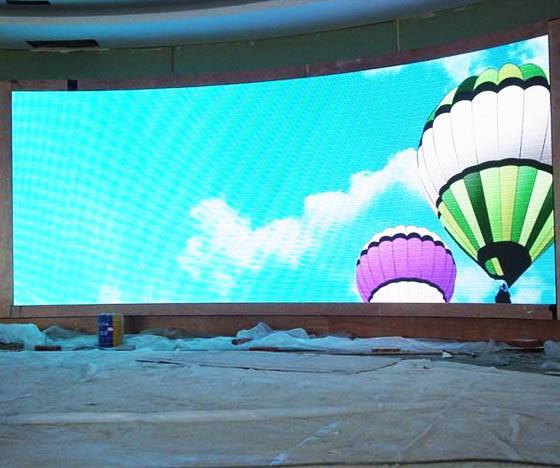How to calculate the arc length and string height of the curved LED display?
1. Measure the length of the string and the length of the arc.
Generally, the arc length is difficult to measure and the size difference is large, so the arc length and radius are calculated according to the chord length of the string, and the check is performed. The difference can be within 10cm. If the difference is large, please measure and calculate repeatedly.
2. String height chord length measurement method.
The straight line distance between the two ends of the arc is the chord length, and the midpoint of the chord length to the midpoint of the curved line is the chord height (or: the midpoint of the chord length, the vertical chord length, the distance from the line to the arc is the chord height) Chord length of the curved screen
3. Calculate the arc length and radius according to the height of the LED display string.
Calculation idea: The root formula calculates the angle between the chord height and the chord length to form a right triangle. example:
1 set the string height to b chord length to 2a measure 2a = 200 b = 60 calculate the arc length and radius
2 string high chord length constitutes the side length of the triangle is a = 100 b = 60 string height chord length calculation method
The length of the three-string high chord is the length of the triangle, which is a=100 b=60. According to the Pythagorean theorem, the formula of the c-side long Pythagorean theorem is calculated: a square + b square = c square is: c = 116.62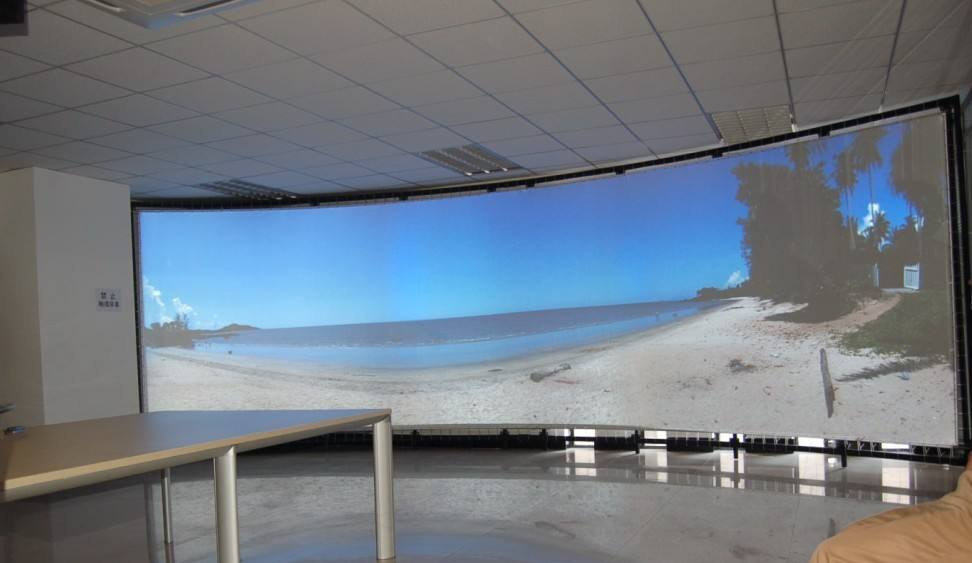4 according to the trigonometric function formula sin âˆ  A = a / c (âˆ  A is the diagonal of a) That is: sin âˆ  A = 0.86 and then use the inverse sine function of the calculator to calculate âˆ  A = 59.32 Â° chord length chord angle calculation method
5 Extend the b side to the center of the circle, and connect the apex of B to the center of the circle. That is, an isosceles triangle is formed. Calculate the arc of the curved screen with a curvature of 124Â° according to the inner angle of the triangle and 180Â° to obtain the isosceles triangle âˆ C=62Â°.
6 According to the formula of sinâˆ C=c/beveled edge, sin62=100/beveled edge and then the sin62 of the calculator is 0.883, that is, the length of the hypotenuse is 113.3 R=113.3 the length of the hypotenuse
7 The circumference of the arc is: circumference = 2Ï€R = 2 * 3.14 * 113.3 = 711.52 circumference / 360, the angle of this circle is: 1.98 arc length = 1.98 per degree value * billboard arc 124 Â° = 245.52.
The final result is arc length: 245.52 radians: 124Â° Radius: 113.3 curved screen calculation method
4. Calculate the arc length of the LED display and compare it with the actual measured arc length
The difference can be within 250px range. If the difference is large, please measure and calculate repeatedly.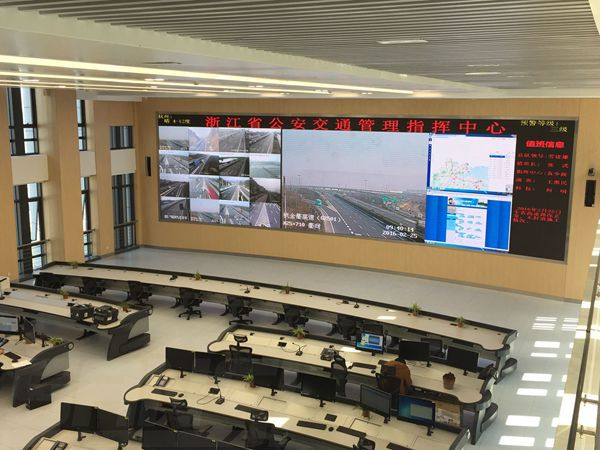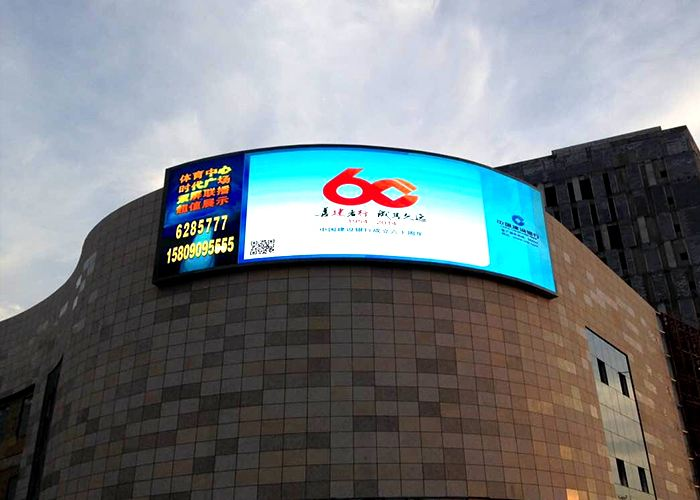Wuhan HAE Uv Flatbed Printer adopt Toshiba Printing and UV ink, Wide Format UV Inkjet Printer can print excellent images on various rigid medias, such as glass, acrylic, wood, PVC foam board, KT board, MDF, alunimum, cellphone case, ceramic tiles, and so on. The Printing Size can be 1325, 3020, 1610 and 9060 for choice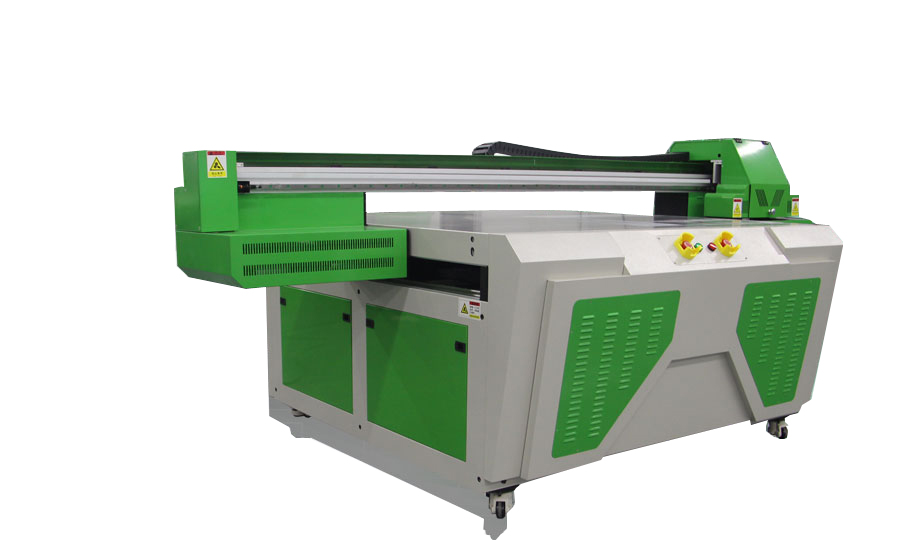UV LED Flatbed Printer 9060

Uv Led Printing Machine,Uv Printing Machine,Uv Led Printer,Wide Format Flatbed Printer

Wuhan HAE Technology Co., Ltd. , https://www.chinahae.com